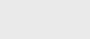2年前 (2021-10-17) |   抢沙发  281

[收起] 文章目录

Java8引入了Lambda形式的函数式编程。术语Lambda来自Lambda演算，用于描述计算。

## Lambda

`(parameters) -> expression`

lambda表达式可以在函数接口的上下文中使用。

## 函数接口

``````public interface Comparator<T> {
int compare(T o1, T o2);
}
public interface Runnable {
void run();
}``````

Lambda表达式允许我们直接内联提供函数接口的抽象方法的实现，并将整个表达式视为函数接口的实例。

## Java函数接口

### Predicate

`Predicate<T>`接口定义了一个名为`test`的抽象方法，该方法接受一个泛型类型为`T`的对象并返回一个布尔值。此接口可用于表示使用T类型对象的布尔表达式。

``````@FunctionalInterface
public interface Predicate<T> {
boolean test(T t);
}``````

### Consumer

`java.util.function.Consumer<T>`接口定义了一个名为`accept`的抽象方法，该方法接受一个泛型类型为`T`的对象，并且不返回任何结果（`void`）。当我们需要访问T类型的对象并对其执行某些操作时，可以使用此接口。

### Function

`java.util.function.function<T，R>`接口定义了一个名为`apply`的抽象方法，该方法将一个泛型类型为`T`的对象作为输入，并返回一个泛型类型为`R`的对象。当我们需要定义一个lambda将信息从输入对象映射到输出时，可以使用该接口。

### Primitive Specializations

``````public interface IntPredicate {
boolean test(int t);
}``````

## 类型检查

lambda的类型是从使用lambda的上下文中推导出来的。上下文中lambda表达式所需的类型（例如，传递给它的方法参数或分配给它的局部变量）称为目标类型。Lambda表达式可以从赋值上下文、方法调用上下文（参数和返回）和强制转换上下文中获取其目标类型。

``Object o = (Runnable) () -> System.out.println("Hello");``

### Capturing Lambda

lambda可以不受限制地捕获（在其主体中引用）实例变量和静态变量。但是当捕获局部变量时，它们必须显式地声明为`final`或实际上是`final`

## 方法引用

• 对静态方法的方法引用。例如，–`Integer:：parseInt`
• 对任意类型的实例方法的方法引用。示例–`String::length`
• 对现有对象或表达式的实例方法的方法引用。示例–`student:：getRank`，其中`student`是具有方法`getRank（）``student`类型的局部变量
``````List<String> list = Arrays.asList("a","b","A","B");
list.sort((s1, s2) -> s1.compareToIgnoreCase(s2));``````

``````List<String> list = Arrays.asList("a","b","A","B");
list.sort(String::compareToIgnoreCase);``````

### 构造函数引用

`Supplier<List<String>> supplier = ArrayList::new`；与`Supplier<List<String>> supplier = () -> new ArrayList<>()`相同；

### 组合Lambda

• 将两个谓词组合成一个较大的谓词，在两个谓词之间执行or操作
• 反向或链式比较器

### Comparators

``````Comparator<Student> c = Comparator.comparing(Student::getRank);
students.sort(comparing(Student::getRank).reversed()); ``````

``````students.sort(comparing(Student::getName).reversed()
.thenComparing(Student::getRank)); ``````

### Predicates

Predicates接口包括三个方法：`negate`, `and`, 和 `or`，可用于创建更复杂的谓词。

``````Predicate<Integer> naturalNumber = i -> i > 0;
Predicate<Integer> naturalNumberLessThanHundred = naturalNumber.and( i -> i < 100);``````

### Functions

`Consider f(x) = x2 and g(x) = x3 + 1 then`

`g(f(x)) ->`

``````Function<Integer,Integer> square = n -> n*n;
Function<Integer,Integer> squareAndCube = square.andThen(n -> n*n*n+1);
System.out.println(squareAndCube.apply(2));
65                        ``````

`f(g(x)) ->`

``````Function<Integer,Integer> square = n -> n*n;
Function<Integer,Integer> squareAndCube = square.compose(n -> n*n*n + 1);
System.out.println(squareAndCube.apply(2));                               ``````

``````public static List<Book> filter(Predicate<Book> where) {
List<Book> books = Catalogue.books();
return books.stream().filter(where).collect(Collectors.toList());
}                                                                       ``````

Lambda表达式通过不同的过滤器过滤不同的书籍

``````List<Book> javaBook = filter(book -> book.getCategory().equals(JAVA));
List<Book> joshuaBlochBook = filter(book -> book.getAuthor().equals("Joshua Bloch"));``````

## 总结

lambda表达式可以被认为是一个匿名函数，可以在函数接口的上下文中使用。函数接口是只指定一个抽象方法的接口。### 注册